## ML Aggarwal Class 6 Solutions for ICSE Maths Model Question Paper 2

Choose the correct answer from the given four options (1-2):
Question 1.
Every composite number has atleast
(a) 1 factor
(b) 2 factors
(c) 3 factors
(d) 4 factors
Solution:
3 factors (c)

Question 2.
Which of the following collections form a set?
(a) Collection of 5 odd prime numbers
(b) Collection of 3 most intelligent students of your class
(c) Collection of 4 vowels in English alphabet
(d) Collection of months of a year having less than 31 days.
Solution:
Collection of months of a year having less than 31 days. (d)

Question 3.
Find the prime factorisation of 1320.
Solution:
1320 = 2 × 2 × 2 × 3 × 5 × 11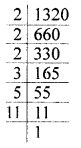Question 4.
If A = {x : x is a positive multiple of 3 less than 20} and B = {x : x is an odd prime number less than 20}, then find n(A) + n(B).
Solution:
A = {3, 6, 9, 12, 15, 18}
B = {3, 5, 7, 11, 13, 17, 19}
n(A) = 6
n(B) = 7
∴ n(A) + n(B) = 13

Question 5.
Reduce the fraction $$\frac{714}{1386}$$ in its simplest form.
Solution: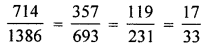Question 6.
Simplify the following:
$$2 \frac{3}{14}-3 \frac{5}{6}-\frac{2}{5}+2 \frac{1}{2}$$
Solution:Question 7.
A number is divisible by 5 and 8 both. By what other numbers with that number be always divisible?
Solution:
1, 2, 4, 10, 20, 40

Question 8.
Arrange the fractions $$\frac{2}{3}, \frac{7}{9}, \frac{5}{8}, \frac{3}{5}$$ in ascending order.
Solution: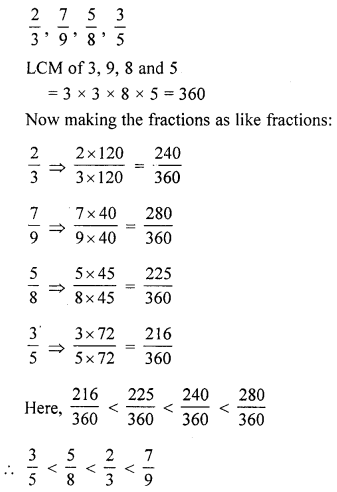Question 9.
Find the smallest number of 5-digits which is divisible by 12, 15 and 18.
Solution:
12, 15 and 18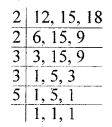∴ LCM of given numbers = 2 × 2 × 3 × 3 × 5 = 180
Smallest number of 5-digits = 10000
We divide 10000 by 180 and find the remainder.According to the given condition,
we need the least number of 5-digits
which is exactly divisible by 12, 15 and 18.
The required number = 10000 + (180 – 100) = 10080

Question 10.
Three bells are ringing continuously at intervals of 30, 40 and 45 minutes respectively. At what time will they ring together if they ring simultaneously at 5 A.M.?
Solution:
LCM = 2 × 2× 2 × 3 × 3 × 5 = 360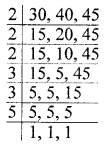After = 360 minutes
360 minutes = 6 hours
They will ring together again at 11 a.m.